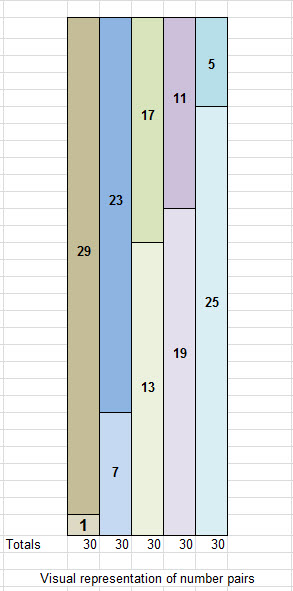Big Prime Number

# Matrix 2

Another set of numbers can be derived from the first matrix members by repetitively adding the number 6 to some of the members of the matrix. It works with the numbers 1 and 5 but this does not work on the number 3. Six is the PF of 2×3. We see that 3 is no longer a seed number for larger primes because it was used to compute the PF. This means a rule is needed to handle a prime number that was used to compute the lower PF number. The first rule is to exclude any prime number greater than 1 from the list of seed numbers that is used to compute the lower PF number for a matrix.

All of the numbers in Matrix 2 are bounded by the lower PF of 6 and the upper PF of 30. It is clear that the number 1 is always going to be part of the list of seed numbers for every single matrix. In this case 1+6 generated 7, 7+6 led to 13, 13+6 produced 19 and 19+6 yielded 25. All of the numbers in the second row can be similarly derived from the seed number 5 by adding 6 repetitively. All of the numbers in this matrix are prime except for the number 25. In Matrix 4, we will see the importance of some compound numbers. The entire matrix and the seed number column is made of number pairs that add-up to the Upper PF number of 30. These include 1+29, 7+23 etc. Below, I show Matrix 2 and the visual representation of these pairs along with Matrix 2. Also, a similar pairing exists in each row. (ex. 1+25 = 7+19 ). You will see that in all the other matrices, there are pairs of numbers in each row that add up to a common number. The common number is only common to the pairs in that row and it changes for each row. However, the extent of the symmetry of these numbers in the matrices is quite extraordinary. The second rule is that every matrix member can be computed by repeatedly adding the lower PF number to a seed number as long as the result is less than the next higher PF.The numbers from Matrix 2 are paired-up in the graphic and show that all of the numbers in the matrix and the seed column are used to yield 5 number pairs. Each pair sums to the Upper PF number of 30. This rather extraordinary symmetry means that numbers relatively prime to a PF occur in the exact same order when counting forward from 1 as they do counting backward from the PF number. This will have significant consequences that will be discussed in later matrices.

In September 2004, I received proof from a mathematician Dr. Jagrata Minardi, that every number relatively prime to an upper PF was the sum of a number relatively prime to the lower PF plus a multiple of the lower PF. In addition the proof showed that there exist no numbers relatively prime to the upper PF that do not satisfy this computation. This statement basically says that the set of numbers generated by the computation includes the entire set of numbers relatively prime to the upper PF. This means we have a bullet proof architectural numeric grid that can be easily computed that holds all prime numbers.

Although I have no mathematical proof regarding the symmetry of paired numbers in the matrix always adding to the upper PF number, it is clearly a fact that holds true for all matrices. If it were not a fact, then the proof provided by Dr. Minardi would not be valid.